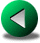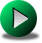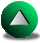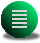Previous: Vorticity Budgets in SW
Next: Discussion and Conclusion
Up: Finite Difference Methods in

Subsections

# The Quasi-Geostrophic Model

## Discretization

We also investigated the influence of coastline discretization in quasi-geostrophic (QG) models, although our main interest in this thesis is focused on the shallow water (SW) models. QG models solve the vorticity equation directly. It seems therefore a reasonable assumption that these models should yield more accurate vorticity budgets than do SW and primitive equation models. The vorticity equation used is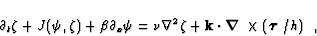(4.24)

where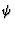is the streamfunction. Equation 4.24 corresponds to a barotropic and geostrophic ocean with a rigid lid approximation. The wind forcing is altered to include the influence of the water depth in order to better mimic the shallow water equations. The discretization of (4.24) is done using second order center differencings. The streamfunction formulation () leads to a linear pentagonal system of equations to solve at each time step. We used the simple leapfrog time integration and the viscous term is discretized by the conventional five-point Laplacian. We are interested in testing different formulations of the Jacobian in (4.24), as the formulation of this term may have consequences for the vorticity budget for the same reasons mentioned previously for the C-grid model.

As for the SW C-grid model, the vorticity budget for the QG model is defined only on an interior sub-domain, half a grid point inside the model basin. This follows from the fact that the vorticity equation is only solved at interior points (see Figure 4.1). The discretized vorticity budget is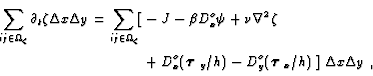(4.25)

where notation is found in Section 2.2.2 and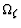is the ensemble of indices for points whose location lies in the interior domain. By defining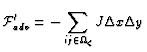(4.26)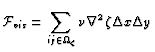(4.27)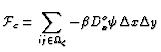(4.28)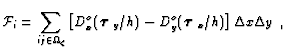(4.29)

we recast the vorticity budget in the following form(4.30)

One main characteristic of QG vorticity budgets is the explicit contribution of the beta term,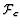. This contribution is hidden in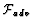for the SW models. Therefore, we definehere to be, where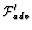represents the integration of the Jacobian term over. We focus our study on the behavior of bothand. As for the C-grid model, a minimum requirement is thatgoes to zero at infinite resolution. This also applies toandseparately. We propose to test three different numerical formulations of the Jacobian, J1, J3 and J7, as termed by Arakawa and Lamb arakawa77 (hereafter, AL77) and investigate their respective influence on the vorticity budget. Other discretization techniques exist that nullify, such as that developed by Salmon and Talley salmon89, but we fear that those techniques miss the point that the vorticity budget can not clearly be defined in the sub-region along the boundaries. Such a technique may reduce the actual size of the model domain to the point that the critical sub-region disappears.

Representation of the Jacobian in (4.25) has been extensively considered by Arakawa arakawa66 and AL77. From the latter, we borrow the notation Ji, where J is the discretized Jacobian and itakes values between 1 to 7, depending on the discretized formulation. The simplest representation is the J1 Jacobian, where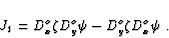(4.31)

J1 conserves relative vorticity in doubly periodic domains, straight channels and rectangular domains when the free-slip boundary condition is applied. In fact,is zero for zero rotation angle becauseandare both zero at the boundary. However, due to its poor conservation properties (energy and enstrophy), other forms of the Jacobian have been suggested.

AL77 proposed the J3 form of the Jacobian which conserves energy in doubly periodic domains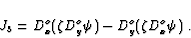(4.32)

The J3Jacobian conserves relative vorticity in doubly periodic domains, but not in presence of boundaries. The boundary terms that arise are relatively easy to pinpoint. They correspond to the value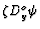or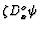at locations one grid point away from the boundaries.

It is interesting to note that the J3 formulation is similar in structure to the advective terms in the SW vorticity equation when the B combination, discussed above, is employed. For example, if we takeand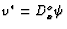, then J3 can be recast as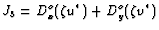. The advective term for the B combination in the vorticity equation takes the form of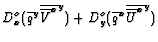. Hence the two formulations use a divergence form of the advection. Moreover, the viscous term in the SW vorticity equation derived using the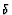-## Results

Using J1, the solutions are very different for positive and negative values of the rotation angle of the basin. Positive angles are characterized by larger kinetic energy and stronger oscillations of a Rossby basin mode (curve b of Figure 4.9), which appears to be unstable at low resolution. However, with increasing resolution (curves d-f of Figure 4.9), the kinetic energy for both positive and negative angles seems to converge to the value of kinetic energy for the non-rotated basin cases (curves a,d). Nonetheless, we prefer to discard this formulation of the Jacobian for the rest of the discussion, due to its low level of accuracy at moderate resolutions.

On the other hand, solutions using J3 and J7 appear stable and converge reasonably well with increasing resolution to the same value of kinetic energy, for both rotated and non-rotated basins (Fig. 4.10). Therefore, this results contrasts with those of the SW model for which the convergence was only obtained for the B combination. The QG model appears to be less sensitive to grid rotations and advective formulations.

In terms of vorticity budget, we are interested in the behavior of the advective contribution,, with increasing resolution for the J3and the J7 Jacobians. Specifically, we are interested in how the convergence order fordiffers in the QG model compared to the SW model. As mentioned,is made of two independent contributions,and.depends directly on the Jacobian formulation butdoes not. Figure 4.11 shows the convergence ofin rotated and non-rotated basins for the two considered Jacobians.is close to second order in non-rotated basins for both Jacobians. At 30o rotation, however, the convergence order is closer to unity for J3 but second order for J7.

We now analyze the convergence order for, the second contribution to. Figure 4.12 shows the convergence forin rotated and non-rotated basins under J3 and J7. The results appear independent of the Jacobian formulation, as expected. The convergence order is however unity, in contrast with results for. This result comes readily from the traditional treatment of the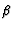term. The proof is given in a square domain: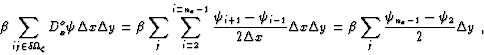(4.34)

since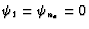, by definition of no-permeability. The west-east asymmetry due to the beta effect imposes that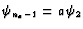with 0< a < 1 and all other parameters kept constant. The factor, a, represents the ratio of the velocity along west and east coastline. Becauseand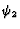converge linearly to zero with increasing resolution, the beta contribution cannot have a better convergence rate than one. In absolute value,is also larger than. Therefore,suffers primarily from the low convergence rate of the beta contribution,. One can ask whether we can get a better convergence order by including the planetary vorticity,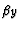in the Jacobian instead of treating it separately (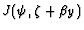instead of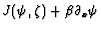). We conducted this experiment with the best advective formulation, the J7. However, convergence order ofis again unity and errors are very similar to the previous case (not shown).

One last point we would like to make is related to similarities mentioned above, between the J3-QG and the B combination of the SW model. Figure 4.13 shows,andwith increasing resolution for J3 and under -30o rotation angle.is negative, goes through an minimum and, then increases toward zero, whereasis positive and decreasing to zero. Hence,appears to go through a pool of negative values, just as the B results showed. This contrasts with results using J7 for whichtakes positive values for both negative and positive rotation angle (not shown).

To conclude, except for the J1 Jacobian, the QG model is less sensitive to the basin rotation, in contrast with results for the SW model. Convergence orders for the advective flux of vorticity,, on the other hand, are order 1 or less--comparable to what was found for the SW simulations. In the QG case, this low order of convergence is related to the beta contribution,. Using J7,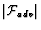varies between 5% (high resolution) and 20% (coarse resolution) of the wind input depending on the rotation angle. These results are somewhat better than those obtained in the SW simulations.Previous: Vorticity Budgets in SW
Next: Discussion and Conclusion
Up: Finite Difference Methods in

Frederic Dupont
2001-09-11